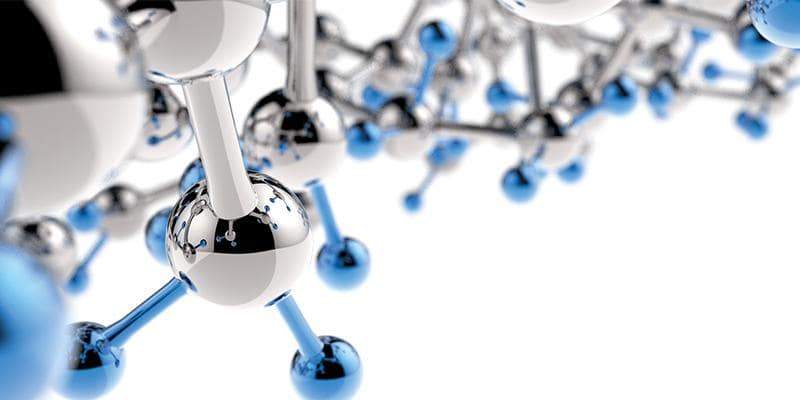Engineering Entrance Sample Papers

Alkaline earth metals Question Bank for JEE MainsQues. To remove last traces of water from alcohol, the metal used is
(a) Sodium
(b) Potassium
(c) Calcium
(d) Aluminium
Ans: (c)

Ques. Setting of plaster of paris is
(a) Oxidation with atmospheric oxygen
(b) Combination with atmospheric CO2
(c) Dehydration
(d) Hydration to yield another hydrate
Ans: (d)

Ques. Calcium is obtained by
(a) Roasting of lime stone
(b) Reduction of CaCl2 with carbon
(c) Electrolysis of a solution of CaCl2 in water
(d) Electrolysis of molten CaCl2
Ans: (d)

Ques. Mortar is a mixture of
(a) CaCO3, sand and water
(b) Slaked lime and water
(c) Slaked lime, sand and water
(d) CaCO3 and CaO
Ans: (c)

Ques. For bleaching powder, which is incorrect
(a) Reacts with dilute acid to release chlorine
(b) Oxidising agent
(c) Light yellow coloured powder
(d) Highly soluble in water
Ans: (d)

Ques. The highly efficient method of obtaining beryllium is
(a) Dissociation of beryllium carbide
(b) Electrolysis of fused beryllium chloride
(c) Reduction of beryllium oxide with carbon
(d) Reduction of beryllium halide with magnesium
Ans: (b)

Ques. Bleaching powder is a compound having the molecular formula
(a) CaOCl3
(b) CaOCl2
(c) CaClO
(d) CaClO3
Ans: (b)

Ques. Electronegativity of beryllium is approximately equal to that of
(a) Aluminium
(b) Boron
(c) Magnesium
(d) Sodium
Ans: (a)

Related: Objective Botany for UAPMT

Ques. Alloys of …… metal are light and strong and so are used in the manufacture of aeroplane parts
(a) Cr
(b) Sn
(c) Fe
(d) Mg
Ans: (d)

Ques. Which of the following alkaline earth metals shows some properties similar to aluminium
(a) Be
(b) Ca
(c) Sr
(d) Ba
Ans: (a)

Ques. A major constituent of portland cement except lime is
(a) Silica
(b) Alumina
(c) Iron oxide
(d) Magnesia
Ans: (a)

Ques. Which of the following decreases on going gradually from Be to Ba (in periodic table)
(a) Basic character of hydroxides
(b) Solubility of sulphates in water
(c) Solubility of hydroxides in water
(d) Strength of elements as reducing agent
Ans: (b)

Ques. Alkaline earth metals come under
(a) Halogens
(b) Representative elements
(c) Transition elements
(d) Inner transition elements
Ans: (b)

Related: VITEEE Physics Sample Papers

Ques. Point out the incorrect statement regarding Be (Group-IIA)
(a) It forms an ionic carbide
(b) Its carbonate decomposes on heating
(c) Its halides are covalent
(d) It is easily attacked by water
Ans: (d)

Ques. Lime stone is
(a) CaO
(b) Ca(OH)2
(c) Both (a) and (b)
(d) None of these
Ans: (d)

Ques. In the Alkaline earth metals, the element forming predominantly covalent compound is
(a) Be
(b) Mg
(c) Sr
(d) Ca
Ans: (a)

Ques. MgCl2 . 6H2O when heated gives
(a) Magnesium oxychloride
(b) Magnesium dichloride
(c) Magnesium oxide
(d) Magnesium chloride
Ans: (c)

Ques. Magnesium does not decompose the
(a) Steam
(b) Hot water
(c) Cold Water
(d) Semi hot water
Ans: (c)

Ques. A metal M readily forms its sulphate MSO4 which is water-soluble. It forms its oxide MO which becomes inert on heating. It forms its insoluble hydroxide M(OH)2 which is soluble in NaOH solution. Then M is
(a) Mg
(b) Ba
(c) Ca
(d) Be
Ans: (d)

Ques. Which is used to reduced the acidity of soil
(a) Calcium hydroxide
(b) Ammonium sulphate
(c) Ammonium nitrate
(d) Ammonium chloride
Ans: (a)

Ques. Li shows the diagonal relationship with
(a) Mg
(b) B
(c) Al
(d) C
Ans: (a)

Ques. Which of the following gives a green colour to flame?
(a) Barium
(b) Calcium
(c) Strontium
(d) None of these
Ans: (a)

Ques. Mg burns in CO to produce
(a) MgO2
(b) MgCO­3
(c) MgO + CO
(d) MgO + C
Ans: (d)

Related: AEEE Sample Papers on Physics

Ques. The number of water molecules in gypsum and plaster of paris respectively are
(a) 1/2 and 2
(b) 2 and ½
(c) 2 and 1
(d) 5 and 2
Ans: (b)